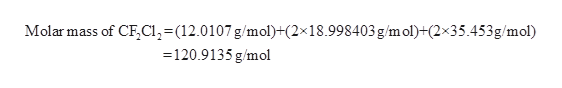How many kilograms of chlorine are in 26 kg of each of the following chlorofluorocarbons (CFCs)?CF2Cl2Express your answer using two significant figures.

Question

How many kilograms of chlorine are in 26 kg of each of the following chlorofluorocarbons (CFCs)?

CF2Cl2
Express your answer using two significant figures.
Step 1

Given,

The compound is CF2Cl2.

Amount of the substance is 26 kg

Step 2

Molar mass of CF2Cl2:help_outlineImage TranscriptioncloseMolar mass of CF,CI,-(12.0107 g/mol)+(2x18.998403 g/m ol)+(2x35.453g/mol) =120.9135 g/mol fullscreen
Step 3

70.906 gram of chlorine is present in 120.9135 grams of CF2Cl2.

Amount of chl...

Want to see the full answer?

See Solution

Want to see this answer and more?

Our solutions are written by experts, many with advanced degrees, and available 24/7

See Solution
Tagged in

General Chemistry